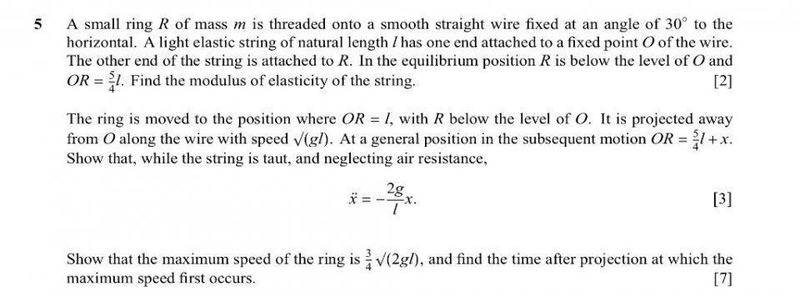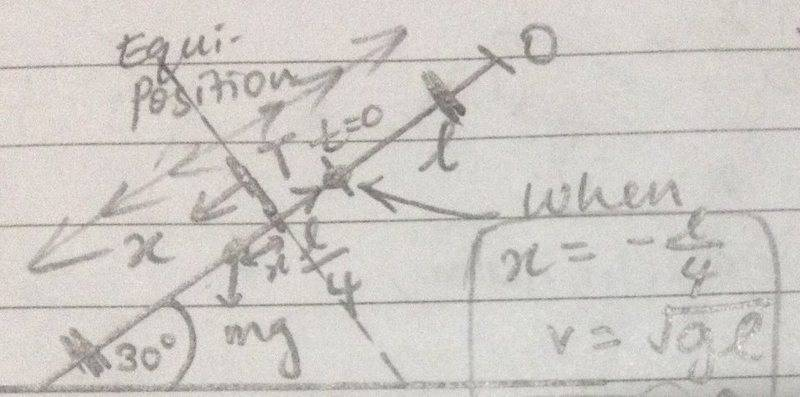# Finding time in a simple harmonic motion

## Homework StatementI seem to have problems finding time in SHM.
To find the time after projection, I know that either x=asinωt or x=acosωt needs to be used, so since R is projected away from O, it means that it is moving towards the equilibrium position, therefore I used x=acosωt to find the time.

## Homework Equations

x=asinωt x=acosωt

## The Attempt at a Solution

x=acosωt
-L/4 = 3L/4 cos (√2g/L)t
t= (√L/2g) cos-1(-1/3)

But, the correct answer should be
t=(√L/2g) sin-1(1/3)

Why can't cosine be used? Since it is moving towards the equilibrium position?

Also, for the equations x=asin(ωt+α) or x=acos(ωt+α)
I am not sure when is α needed to take into consideration.
So far, I never encountered any questions needing to find α, but I would like to know when is α needed.

Thanks very much in advance!

## Answers and Replies

Dr. Courtney
Education Advisor
Gold Member
2020 Award
Drawing a picture always helps.

Drawing a picture always helps.
Yeah I drew a diagramBut I am confused on when to use sine or cosine in the displacement formula..

In choosing sin or cos, do you want the displacement to be a maximum or a minimum at t = 0?
Either to can be converted to the other by including a phase angle of pi / 2.
Here it appears that the displacement is decreasing at t = 0 so it may be that
the cos might be a starting point.

In choosing sin or cos, do you want the displacement to be a maximum or a minimum at t = 0?
Either to can be converted to the other by including a phase angle of pi / 2.
Sin when displacement is a minimum and cos when displacement is a maximum at t=0.

But when t=0, the displacement is -L/4 which is not the amplitude (3L/4), and since it's moving towards the equilibrium position, why not cos?

Isn't mass m being projected from x = - L / 4.
It appears from the wording that L = constant and that x is the displacement from the new equilibrium position at 5 L / 4.
How did you get 3 L / 4 for the amplitude?

Isn't mass m being projected from x = - L / 4.
It appears from the wording that L = constant and that x is the displacement from the new equilibrium position at 5 L / 4.
How did you get 3 L / 4 for the amplitude?
Yeah
v22(a2-x2)
x is the displacement down the slope from the new equilibrium position which is at 5L/4 from O,
When x= -L/4, v=√gL
So, gL = 2g/L (a2-(-L/4)2)
∴ a=3L/4

But, if the particle is displaced at x=-L/4, then it is moving towards the equilibrium position, but it seems that cosine can't be used but sine .
Or has the equilibrium position changed to a distance L from O? Is that why it is sine?

Isn't the displacement increasing at t = 0?
Also, it appears that a phase angle is involved since the displacement
is not zero at t = 0.

Isn't the displacement increasing at t = 0?
Also, it appears that a phase angle is involved since the displacement
is not zero at t = 0.
Sorry, I'm a little confused.. from which point do you define your displacement from? Is it at a distance L or 5L/4 from O?

The problem states that the new equilibrium point is 5 L / 4 and the ring is
moved to distance L so the initial displacement must be (L - 5 L / 4) = - L / 4.
The problem also states that the general position is OR = 5 L / 4 + x which
means that x is the displacement variable and at t = 0, x = - L / 4.
Since the ring is projected away from O, the displacement variable is increasing towards
positive values.
Choosing the sine function fits this description since the sine is also increasing at t = 0.
Note that the speed is also increasing which is described by the cosine function, and the acceleration
in this case would be described by the negative sin function since the restoring force will be
decreasing as the ring moves towards equilibrium at 5 L / 4 as measured from O.
The cosine function can be increasing at t = 0 if the phase angle is negative.
Examining the initial conditions will help in choosing sin or cos to describe the resulting motion.

The problem states that the new equilibrium point is 5 L / 4 and the ring is
moved to distance L so the initial displacement must be (L - 5 L / 4) = - L / 4.
The problem also states that the general position is OR = 5 L / 4 + x which
means that x is the displacement variable and at t = 0, x = - L / 4.
Since the ring is projected away from O, the displacement variable is increasing towards
positive values.
Choosing the sine function fits this description since the sine is also increasing at t = 0.
Note that the speed is also increasing which is described by the cosine function, and the acceleration
in this case would be described by the negative sin function since the restoring force will be
decreasing as the ring moves towards equilibrium at 5 L / 4 as measured from O.
The cosine function can be increasing at t = 0 if the phase angle is negative.
Examining the initial conditions will help in choosing sin or cos to describe the resulting motion.
Thanks, I've got it